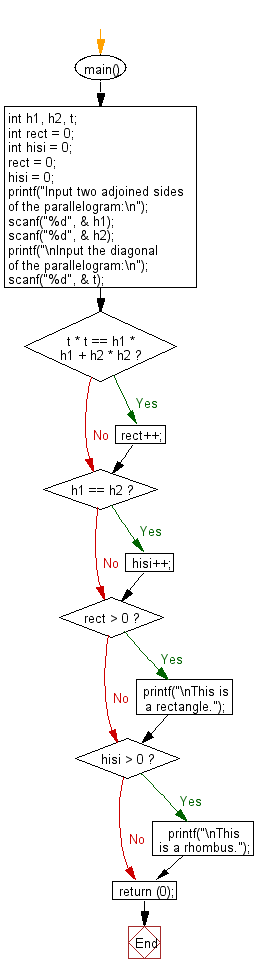﻿ C Program: Check a parallelogram is a rectangle or a rhombus - w3resource

# C Exercises: Check a parallelogram is a rectangle or a rhombus

## C Basic Declarations and Expressions: Exercise-142 with Solution

Write a C program that reads the two adjoining sides and the diagonal of a parallelogram and checks whether the parallelogram is a rectangle or a rhombus.

According to Wikipedia-
parallelograms: In Euclidean geometry, a parallelogram is a simple (non-self-intersecting) quadrilateral with two pairs of parallel sides. The opposite or facing sides of a parallelogram are of equal length and the opposite angles of a parallelogram are of equal measure.
rectangles: In Euclidean plane geometry, a rectangle is a quadrilateral with four right angles. It can also be defined as an equiangular quadrilateral, since equiangular means that all of its angles are equal (360°/4 = 90°). It can also be defined as a parallelogram containing a right angle.
rhombus: In plane Euclidean geometry, a rhombus (plural rhombi or rhombuses) is a simple (non-self-intersecting) quadrilateral whose four sides all have the same length. Another name is equilateral quadrilateral, since equilateral means that all of its sides are equal in length. The rhombus is often called a diamond, after the diamonds suit in playing cards which resembles the projection of an octahedral diamond, or a lozenge, though the former sometimes refers specifically to a rhombus with a 60° angle (see Polyiamond), and the latter sometimes refers specifically to a rhombus with a 45° angle.
Input:
Two adjoined sides and the diagonal.
1 <= ai, bi, ci <= 1000, ai + bi > ci

Sample Solution:

C Code:

``````#include <stdio.h>

int main() {
int h1, h2, t;
int rect = 0;
int hisi = 0;

// Initialize variables
rect = 0;
hisi = 0;

// Input the adjoined sides of the parallelogram
printf("Input two adjoined sides of the parallelogram:\n");
scanf("%d", &h1);
scanf("%d", &h2);

// Input the diagonal of the parallelogram
printf("\nInput the diagonal of the parallelogram:\n");
scanf("%d", &t);

// Check if it's a rectangle
if (t * t == h1 * h1 + h2 * h2)
rect++;

// Check if it's a rhombus
if (h1 == h2)
hisi++;

// Output the results
if (rect > 0)
printf("\nThis is a rectangle.");
if (hisi > 0)
printf("\nThis is a rhombus.");

return 0;
}
``````

Sample Output:

```Input two adjoined sides of the parallelogram:
3
4

Input the diagonal of the parallelogram:
5

This is a rectangle.
```

Sample Output:

```Input two adjoined sides of the parallelogram:
5
5

Input the diagonal of the parallelogram:
7

This is a rhombus.
```

Flowchart:C programming Code Editor:

What is the difficulty level of this exercise?

Test your Programming skills with w3resource's quiz.

﻿